# Recognition and Analysis of Behavior Features of School-Age Children Based on Video Image Processing

Recognition and Analysis of Behavior Features of School-Age Children Based on Video Image Processing
School of Education, Xi'an Fanyi University, Xi'an 710105, China

Corresponding Author Email:
Alfrent123@163.com
Page:
603-610
|
DOI:
https://doi.org/10.18280/ts.370408
29 March 2020
|
Accepted:
2 July 2020
|
Published:
10 October 2020
| Citation

OPEN ACCESS

Abstract:

School-age children have vastly different behavior features from adults. Most of the relevant studies are theoretical summaries of behavior features of these children, failing to detect the behaviors or recognize the behavior features in an accurate manner. To solve the problem, this paper puts forward a novel method to recognize the behavior features of school-age children through video image processing. Firstly, the authors designed a method to extract static behavior features of school-age children from surveillance video images. Next, the behavior features of school-age children were extracted by optical flow method. On this basis, a dual-network flow neural network (DNFNN) was designed, in which the time flow network processes the dense optical flow of multiple continuous frames of the surveillance video, while the spatial flow network treats the region of interest (ROI) in the static frame from the video. After that, the workflow of the DNFNN was introduced in details. Experimental results fully demonstrate the effectiveness of the proposed network. The research findings provide a reference for the application of video image processing to behavior recognition in other fields.

Keywords:

video image processing, school-age children, behavior features, behavior recognition

1. Introduction

The behaviors of adults have fixed patterns and take place in regular locations. In contrast, the behaviors of school-age children carry some distinctive features [1-4]. The daily activities of school-age children often take place in open spaces like schools and streets. While undergoing rapid mental and physical growth, school-age children are prone to be injured, due to their immature and unstable state of mind. To safeguard the healthy growth of school-age children, it is of great significance to explore the space and laws of their behaviors, with full consideration of their mental needs and physiological scale. The proliferation of video surveillance system makes it possible to recognize, analyze, and summarize the behavior features of school-age children, with the aid of advanced image processing technology [5-9].

Some scholars have theoretically summarized the behavior features of school-age children [10-14]. For instance, Maramis et al.  defined the features of children behaviors, constructed three behavior patterns (i.e. essential behavior, spontaneous behavior, and social behavior) of school-age children in five aspects (i.e. mode, behavior, relationship, requirement, and location), and identified relevance as the most striking behavior feature of school-age children, that is, each behavior pattern is usually derived from the other two patterns. Mabrouk and Zagrouba  explored the relationship between the safety of children behavior space and children behavior features, and provided the safety analysis and design methods of playground design. Zhang et al.  classified and summarized the cognitive behaviors of preschool children, and refined their behavior features in four dimensions (i.e. cognitive behavior, perception behavior, action behavior and social behavior), from the angle of sensory integration.

Moving target detection is the basis for recognizing the behavior features of school-age children based on video images. Most of the relevant studies focus on moving target extraction algorithms, namely, frame difference method and background modeling [15, 17-19]. Kennedy et al.  optimized the moving foreground detection algorithm in the case of camera shake, and updated the detection strategy by treating the background with first-in, first-out method and setting an adaptive threshold, thereby improving the adaptability to scene changes. Cord  combined nonparametric human moving target estimation and image registration method to generate background images with the same perspective as the background, while extracting the foreground. Cao et al.  developed an incremental activity learning framework that can continuously update the human activity model and renew the learning model from unknown videos, providing a solution to the manual labeling of the samples learned from different kinds of human behaviors. Sandler et al.  proposed a human behavior detection method, which relies on local binary similarity to extract foreground targets and joint features, and classified the behaviors of monitoring targets by fusing the joint features with histogram of oriented gradients (HOG) features and pyramid features.

In the open outdoor environment, there is a heavy presence of interference, which may induce errors in the behavior detection and recognition of school-age children. Technically, it is an urgent problem to guarantee the recognition accuracy of the behavior features of school-age children, despite the growing maturity of technologies like artificial intelligence (AI) and data mining, as well as the rising accuracy and speed of image feature extraction and moving target detection algorithms.

Based on video image processing, this paper puts forward a novel method to recognize the behavior features of school-age children. Firstly, the authors designed a method to extract static behavior features of school-age children from surveillance video images. Then, the movement features of school-age children were extracted by optical flow method. On this basis, a dual-network flow neural network (DNFNN) was designed, in which the time flow network processes the dense optical flow of multiple continuous frames of the surveillance video, while the spatial flow network treats the region of interest (ROI) in the static frame from the video. The workflow of the DNFNN was introduced in details. Finally, the proposed network was proved valid through experiments.

2. Extraction of Static Features

To detect the behaviors of school-age children, the first step is the moving target detection in video images. The detection effect directly bears on the extraction accuracy of behavior features. Compared with the traditional background modeling algorithm, the ViBe algorithm is good at simulating the stochastic changes of pixels. But the algorithm might make false detection, under the influence of image background and ghosting. In this paper, the ViBe algorithm is improved from the perspective of pixel classification threshold (PCT). Firstly, the background complexity in the static frame from the video was defined as:

$\varepsilon =\frac{\alpha }{h\times w}\sum\limits_{k=1}^{K}{\sum\limits_{a=0}^{h-1}{\sum\limits_{b=0}^{w-1}{\left| p(x)-{{p}_{k}}(x) \right|}}}$           (1)

where, p(x)-pk(x) is the difference between a pixel in the current frame and the corresponding pixel in an image from the sample set of the background model; α is the suppression coefficient reflecting the degree of change of video image background. Let ∇T be the degree of change of the background. Then, the PCT that adapts to the change of ∇T can be expressed as:

T(x)=\left\{ \begin{align} & T(x)(1-\beta ),T(x)>\varepsilon \\ & T(x)(1+\beta ),T(x)\le \varepsilon \\ \end{align} \right.           (2)

where, β is the adjustment variable of fixed threshold. To rapidly adjust the change speed of PCT with the change of ∇T, β should be adjusted by:

\beta =\left\{ \begin{align} & \frac{T(x)-\varepsilon }{\varepsilon },T(x)>\varepsilon \\ & \frac{\varepsilon -T(x)}{\varepsilon },T(x)\le \varepsilon \\ \end{align} \right.               (3)

Substituting (3) into (4), the PCT can be expressed as:

T(x)=\left\{ \begin{align} & T(x)(1-\frac{T(x)-\varepsilon }{\varepsilon }),T(x)>\varepsilon \\ & T(x)(1+\frac{\varepsilon -T(x)}{\varepsilon }),T(x)\le \varepsilon \\ \end{align} \right.         (4)

Simplifying (4), the adaptive PCT can be expressed as:

$T(x)=T(x)(2-\frac{T(x)}{\varepsilon })$           (5)

To prevent misjudgment of the adaptive PCT, the threshold should be adjusted back to 1.5∇T, if the adaptive threshold reaches or surpasses 2∇T.

On the extraction of moving target features, speeded up robust features (SURF) is much simpler than the scale-invariant feature transform (SIFT). When it comes to the extraction of interesting feature points from video images, the Hessian matrix of SURF needs a complex calculation process and a long time to filter out the incorrect points of interest (POIs).

To solve the above defect and suppress noise interference, this paper introduces the similar pixel-based response function correction factor to the Harris corner detection. In the input video image, the similarity between the target pixel p(a1,b1) and any pixel p(a2,b2) in its neighborhood can be calculated by:

$S(a,b)=\sum\limits_{a=0}^{h-1}{\sum\limits_{b=0}^{w-1}{s(a,b)}}$              (6)

where, s(a,b) is a binary function representing the relationship between the absolute value of the grayscale difference of the two pixels and the set threshold. If the absolute value is smaller than the set threshold, s(a,b)=1; otherwise, s(a,b)=0.

## 1.png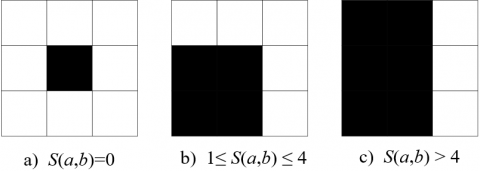Figure 1. The similarity values in three cases

Figure 1 shows the similarity values in three cases: (a) an isolated point or a noise point; (b) candidate corners; (c) edge points of the target. The gradients of the selected candidate corners can be multiplied horizontally and vertically by:

$M_{a}^{2}={{M}_{a}}\cdot {{M}_{a}}=\frac{\partial p}{\partial a}\cdot \frac{\partial p}{\partial a}={{\left\langle M\otimes [-1\text{ }0\text{ }1] \right\rangle }^{\text{2}}}$          (7)

$M_{b}^{2}={{M}_{b}}\cdot {{M}_{b}}=\frac{\partial p}{\partial b}\cdot \frac{\partial p}{\partial b}={{\left\langle M\otimes {{[-1\text{ }0\text{ }1]}^{T}} \right\rangle }^{2}}$                (8)

\begin{align} & {{M}_{ab}}={{M}_{a}}\cdot {{M}_{b}}=\frac{\partial p}{\partial a}\cdot \frac{\partial p}{\partial b} \text{ }=\left\langle M\otimes [-1\text{ }0\text{ }1] \right\rangle \cdot \left\langle M\otimes {{[-1\text{ }0\text{ }1]}^{T}} \right\rangle \\ \end{align}             (9)

The matrix form of the gradient product can be weighted by the Gaussian function below:

{{M}_{mat}}=\left[ \begin{align} & \sum\limits_{\omega }{M_{a}^{2}\text{ }\sum\limits_{\omega }{{{M}_{a}}{{M}_{b}}}} \\ & \sum\limits_{\omega }{{{M}_{a}}{{M}_{b}}\text{ }\sum\limits_{\omega }{M_{a}^{2}}} \\ \end{align} \right]             (10)

where, ω is the weight coefficient of pixel (a,b). The Harris function of each pixel can be calculated by:

$H=\det ({{M}_{mat}})-\delta {{\left[ \text{tra}({{M}_{mat}}) \right]}^{2}}$                  (11)

where, det(Mmat) and tra(Mmat) are the product and the sum of the eigenvalues of matrix Mmat, respectively; δ is an empirical constant in the range of 0.04~0.06. The above formula can be modified with a correction factor:

${{H}^{*}}=\frac{\text{1}}{S(a,b)}\times H,S(a,b)\in [1,4]$              (12)

The POI or the final corner of SURF can be determined through non-maximum suppression by (12).

3. Extraction of Movement Features

To a certain extent, the brightness of video images reflects the movements of school-age children. Hence, the optical flow was chosen to characterize the movement features of these children. Let g(a,b,t) be the grayscale of pixel (a,b) in a video frame at time t. Then, the Taylor formula can be derived from the functions of the pixel’s position and time:

\begin{align} & F(a+da,b+db,t+dt) \text{ }=F(a,b,t)+{{F}_{a}}da+{{F}_{b}}db+{{F}_{t}}dt+\Delta ({{\upsilon }^{2}}) \\ \end{align}                  (13)

where, Fa, Fb, and Ft are the partial derivatives of F in directions a, b, and t, respectively. If pixel (a,b) moves a distance of (da,db) in a tiny period Δt, and if the pixel brightness does not vary with time, then the following equation holds:

$F(a+da,b+db,t+dt)=F(a,b,t)$                 (14)

If da, db, and dt are infinitely small, then $\Delta\left(v^{2}\right)$ in (13) is a negligible second-order infinitesimal. Thus, the optical flow equation can be expressed as:

$-{{F}_{t}}={{F}_{a}}\frac{da}{dt}+{{F}_{b}}\frac{db}{dt}$          (15)

Let v and u be the velocity vectors of the optical flow along the X and Y axis, respectively. Then, the optical flow feature (v,u) is the required optical flow vector. After estimating the movement features of school-age children by the optical flow method, the video frame can be divided into several blocks along the width and height. Figure 2 shows the workflow of histogram statistics for multi-scale optical flows.

## 2.png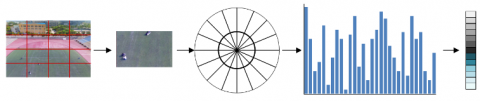Figure 2. The workflow of histogram statistics for multi-scale optical flows

## 3a.png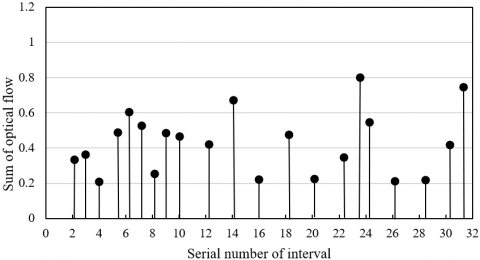(a)

## 3b.png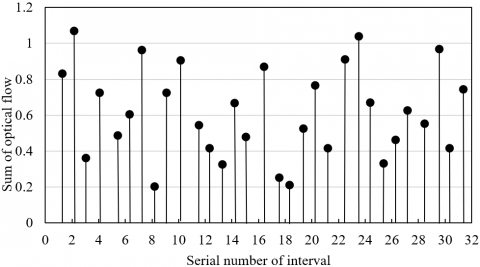(b)

Figure 3. The multi-scale optical flow histograms of adults (a) and school-age children (b)

First, the entire frame was divided into several fixed-size blocks. Based on the angle of the optical flow, each block was further divided into q intervals of the size 2π/q. As shown in Figure 2, the video frame is split into 16 80×80 blocks, each of which is divided into 16 intervals of 22.5°.

According to the size of the optical flow, all the intervals were categorized into 2 scales. The first 16 intervals correspond to the 8 directions in which the inner optical flow is smaller than the set threshold Δω, while the last 16 intervals correspond to the 8 directions in which the outer optical flow is greater than that threshold.

The interval division of pixel (a, b) can be expressed as:

W(a,b)=\left\{ \begin{align} & r(\frac{qR(x,y)}{2\pi })\bmod q,V\text{(}x,y\text{)}<\Delta \omega \\ & r(\frac{qR(x,y)}{2\pi })\bmod q+q,V\text{(}x,y\text{)}\ge \Delta \omega \\ \end{align} \right.               (16)

where, W(a,b) is the serial number of histogram intervals; V(a,b) and R(a,b) are the velocity and direction of the optical flow at pixel (x,y), respectively. After the classification of the optical flow, the sum of optical flow in each interval was counted and taken as the height of the histogram of that interval.

Figures 3 (a) and (b) are the multi-scale optical flow histograms derived from normal samples of adults and school-age children, respectively. It can be seen that the optical flow amplitude in each interval of adults was smaller than that of school-age children. This is because the main movement directions of adults are more uniform than those of school-age children.

4. DNFNN Construction

To effectively recognize the behaviors of school-age children in the set of multiple video frames, this paper puts forward a DNFNN architecture based on time flow network and spatial flow network. Specifically, the time flow network processes the dense optical flow of multiple continuous frames, to acquire the movement features of school-age children from the surveillance video; the spatial flow network treats the ROI in the static frame from the video, to obtain static information like background and appearance.

To recognize the typical behaviors of school-age children, the spatial flow network is a convolutional neural network (CNN) that extracts the features of each video frame, and mines the static information (e.g. background and appearance) from RGB (red, green, blue) imageS by the principle of image recognition. A shown in Figure 4, the spatial CNN is an improved 8-layer VGGNet, where max pooling and rectified linear unit (ReLU) activation function are adopted on each layer.

## 4.png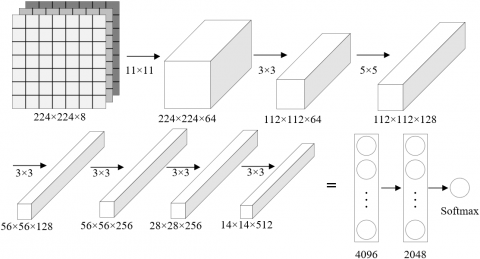Figure 4. The improved VGGNet

## 5.png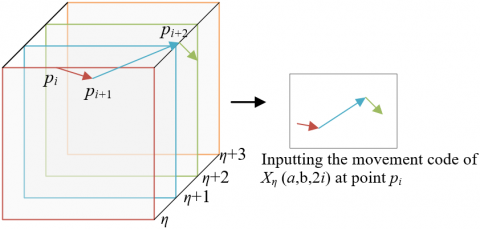Figure 5. The sketch map of trajectory superposition

The time flow network is a temporal CNN that mainly extracts the optical flow containing the movements or time series information of the behaviors of school-age children. Here, the optical flow signals are inputted through trajectory superposition (Figure 5). The pixels at the same position of continuous frames were sampled, and the movement trajectories were adopted to track the superposed optical flows. For any frame η, the input Xη can be expressed as:

$\left\{ \begin{matrix} {{X}_{\eta }}(a,b,2i-1)=o_{\eta +i-1}^{x}({{p}_{i}}) \\ {{X}_{\eta }}(a,b,2i)=o_{\eta +i-1}^{y}({{p}_{i}}) \\\end{matrix} \right.$                  (17)

where, oη+i+1x and oη+i+1y are the vector fields of the horizontal and vertical components on the η+i+1-th frame, respectively. a=[1,w], b=[1,h], and i=[1,L] for any pixel (a,b); pi is the k-th point along the trajectory of the L sequence frames, which starting from the position of pixel (a,b) in the η-th frame. The value of pi can be defined by the recursive relationship below:

$\left\{ \begin{matrix} {{p}_{1}}=(a,b) \\ {{p}_{i}}={{p}_{i+1}}+{{o}_{\eta +i-2}}({{P}_{k-1}})\text{ ,} i>1 \\\end{matrix} \right.$                  (18)

The DNFNN is implemented in the following steps:

Step 1. Under the framework of the input layer, the image size is uniformly scaled to 224*224; the RGB images are imported to the spatial flow network, and 8 consecutive frames of superposed optical flow images are imported to the time flow network.

Step 2. In the first convolutional layer conv1, the spatial flow network and time flow network convolute the ROIs of the motions of school-age children and the 8 consecutive frames of optical flow images, which are obtained in the previous step. In this way, 64 salient features can be extracted. Let λ be the filling size, 1 be the filling parameter, and snuc be the kernel size. Then, the number of 224*224 feature maps output by the convl can be calculated by:

$O=\frac{I-{{s}_{nuc}}+2\times \lambda }{l}+1$              (19)

where, I and O are the size of the input and output video frames, respectively; l is the step length. The final output of conv1 is 64 224*224 feature maps.

Step 3. In the first pooling layer, the window size is 2×2, and the step length is 2. This layer performs max pooling of the 64 feature maps, changing their size to 112*112.

Step 4. The second convolutional layer conv2 further convolutes the pooled feature maps into 128 112*112 feature maps, using the same kernel size as conv1.

Step 5. Using the same window size, the second pooling layer performs max pooling of the 128 feature maps, changing their size to 56*56.

Step 6. The same convolution and pooling parameters are adopted in the subsequent convolutional and pooling layers. The third convolutional layer conv3 convolutes the 128 pooled feature maps, producing 256 56*56 feature maps. These feature maps are adjusted to the size of 28*28 through max pooling. Next, the fourth convolutional layer conv4 convolutes the 256 pooled feature maps into 512 28*28 feature maps. These feature maps are adjusted again to the size of 14*14 through max pooling.

Step 7. The 512 14*14 feature maps are imported to two fully-connected layers, generates a 4,096-dimensional eigenvector and a 2,048-dimensional eigenvector. The 2048-dimensional vector, as the final representation of movement features of school-age children, is imported to the long short-term memory (LSTM) network, which recursively learns all the long-term movement features in the time dimension. Finally, the softmax outputs of spatial and time flow networks are weighted and merged to obtain the classification results of school-age children’s behaviors.

After the LSTM processing, the output of the last layer was connected to the softmax classifier, and the proposed network was trained by the weighted and merged high-level features. The output of the classifier is usually an N-dimensional vector Cμ(⁎), reflecting the probability for the current input to fall into each class. Let μ be the learning parameter. Then, the classifier output can be normalized by:

\begin{align} & {{C}_{\mu }}(y_{i}^{M})=\left[ \begin{matrix} d(y=1|y_{i}^{M},\mu ) \\ ... \\ d(y=N|y_{i}^{M},\mu ) \\ \end{matrix} \right] \text{ }=\frac{1}{\sum\limits_{j=1}^{K}{\exp ({{\mu }_{j}}y_{i}^{H})}}\left[ \begin{matrix} \exp ({{\mu }_{1}}y_{i}^{H}) \\ ... \\ \exp ({{\mu }_{N}}y_{i}^{H}) \\ \end{matrix} \right] \\ \end{align}              (20)

The deviation of the predicted probability distribution from the actual result can be evaluated by the cross-entropy loss:

\begin{align} & Loss= -\frac{1}{H}\left[ \sum\limits_{i=1}^{H}{{{h}^{(i)}}\log ({{C}_{\mu }}({{y}^{(i)}}))+(1-{{h}^{(i)}})\log (1-{{C}_{\mu }}({{y}^{(i)}}))} \right] \\ \end{align}           (21)

The greater the cross-entropy loss, the larger the deviation.

Rather than take the mean output of softmax classifier as the final prediction, the recognition effect of the DNFNN on school-age children’s behaviors was optimized by the weighting method below:

${{\hat{y}}_{t}}=\arg \max \left[ \tau {{P}_{s}}(t)+(1-\tau ){{P}_{t}}(t) \right]$         (22)

where, τ∈[0,1] is the weight coefficient of the spatial flow network; Ps(ω) and Pt(ω) are the output probabilities of spatial flow network and time flow network, respectively.

5. Experiments and Results Analysis

This paper mainly aims to identify the behavior features of school-age children in video images. Therefore, a surveillance video dataset of a school playground was chosen for our experiments. The dataset contains 50 frame sequences (frame rate: 35fps; resolution: 720*480). Each video clip covers several kinds of behaviors of school-age children, including general behaviors (e.g. walking, running, and jumping), interactive behaviors (e.g. handshaking, pointing, and hugging), and uncivilized behaviors (e.g. hitting, pushing, and kicking).

## 6.png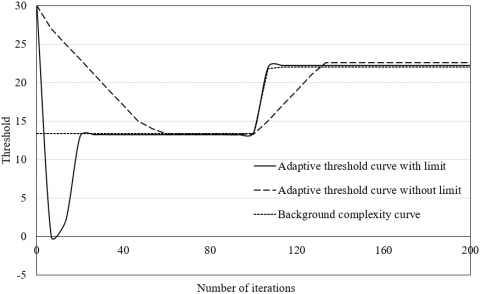Figure 6. The adaptive threshold curves

## 7a.png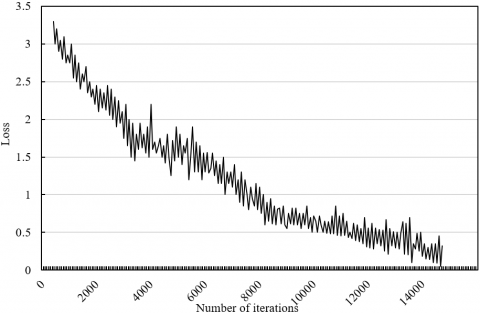(a)

## 7b.png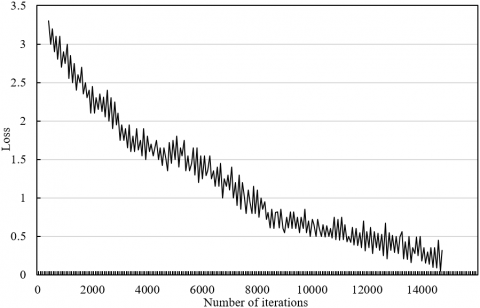(b)

## 7c.png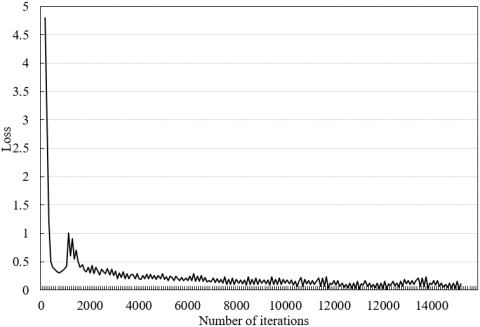(c)

Figure 7. The loss curves of spatial flow network (a), time flow network (b), and DNFNN (c)

In the proposed network, a limit is imposed on the adaptive threshold to enhance the adaptability to the changing background complexity, during the extraction of static features from video images. Figure 6 compares the adaptive threshold curves with and without the limit. It can be seen that, when the background complexity changed suddenly, the limited adaptive threshold converged faster and better than the original adaptive threshold.

Figure 7 presents the loss curves of spatial flow network, time flow network, and DNFNN in the training process. With the growing number of iterations, the losses, i.e. training errors, of all three networks continued to decline, and the predicted classes of the behavior features of school-age children gradually approximated the actual classes. The loss functions of spatial flow network and time flow network converged ideally after about 14,000 iterations; the loss function of the DNFNN also tended to be stable at around the 14,000-th iteration.

## 8.pngFigure 8. The error curves at 8 and 16 optical flow intervals

## 9.pngFigure 9. The recognition accuracies of spatial flow network and time flow network

In our method, different video frames are divided into different number of optical flow intervals. Figure 8 compares the root mean square errors (RMSE) curves at 8 and 16 optical flow intervals. Obviously, the RMSE was smaller at 16 intervals than that at 8 intervals. The greater the number of intervals, the richer the semantics being mined, and the better the recognition effect of the behavior features of school-age children.

The next is to verify the necessity of the merge between the two networks and the adoption of the LSTM network, and to provide the basis for the weight setting during the merge. First, the recognition accuracies of spatial flow network and time flow network are compared in Figure 9. It can be seen that the recognition accuracies of spatial flow network and time flow network fluctuated about 83% and 85%, respectively. The mean accuracies reached 84.5%. The time flow network achieved slightly better recognition effect on the behavior features of school-age children.

Figure 10(a) compares the recognition errors of school-age children’s behaviors with and without LSTM. It can be seen that, the LSTM processing lowered the recognition error in the first 10,000 iterations, because the network fully mines the spatiotemporal information from the video frames.

Considering its slightly better recognition effect than spatial flow network, the time flow network is given the greater weight in our method. To optimize the weight setting, Figure 10(b) compares the recognition accuracies, when the time flow network has different weights. With the growing weight of the time flow network, the recognition accuracy gradually increased; when the weight reached 0.7, the recognition accuracy reached the peak value; further growth of the weight caused the accuracy to drop. The reason is that the optical flows contain rich information about the movement time sequence of school-age children. The superposed optical flow can enhance the movement trend, enabling our network to learn more salient features of behaviors.

## 10a.png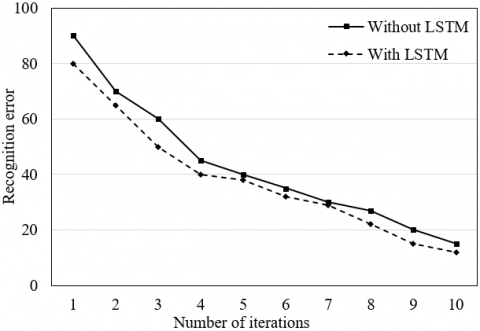(a)

## 10b.png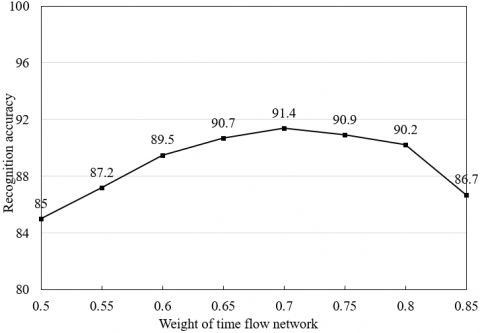(b)

Figure 10. The effects of LSTM (a) and weight setting (b) on recognition effect

6. Conclusions

Based on video image processing, this paper puts forward a novel method to recognize the behavior features of school-age children. Firstly, the authors designed a method to extract static behavior features of school-age children from surveillance video images, and suggested extracting the movement features of these children by optical flow method. Experimental results prove that the two methods can adapt well to images with different background complexities. Next, the spatial flow network was merged with the time flow network into the DNFNN, and the workflow of the DNFNN was introduced in details. Through experiments, it is observed that the DNFNN tended to be stable after about 14,000 iterations, and the predicted classes of the behavior features of school-age children gradually approximated the real classes, with the growing number of training iterations. The experiments also demonstrated the necessity to merge the spatial and time flow networks and adopt the LSTM network, and provided the basis for weight setting for the merge.

Acknowledgements

This paper was supported by the Shaanxi Provincial Department of Education Project (Grant No.: 19JK0343); the Part of the research results of the 2018 school-level teaching team "Kindergarten Management" of Xi’an Fanyi University (Grant No.: T1805); the Online Course Construction Project of Xi'an Fanyi University-Kindergarten and Primary School Class Management (Grant No.: ZK2019).

References

 Sanner, C.M., Neece, C.L. (2018). Parental distress and child behavior problems: Parenting behaviors as mediators. Journal of Child and Family Studies, 27(2): 591-601. https://doi.org/10.1007/s10826-017-0884-4

 Dennis, M.L., Neece, C.L., Fenning, R.M. (2018). Investigating the influence of parenting stress on child behavior problems in children with developmental delay: The role of parent-child relational factors. Advances in Neurodevelopmental Disorders, 2(2): 129-141. https://doi.org/10.1007/s41252-017-0044-2

 Park, K., Kihl, T., Park, S., Kim, M.J., Chang, J. (2016). Narratives and sensor driven cognitive behavior training game platform. 2016 IEEE 14th International Conference on Software Engineering Research, Management and Applications (SERA) - Towson, MD, USA, pp. 125-131. https://doi.org/10.1109/SERA.2016.7516137

 Samad, M.D., Diawara, N., Bobzien, J.L., Harrington, J.W., Witherow, M.A., Iftekharuddin, K.M. (2017). A feasibility study of autism behavioral markers in spontaneous facial, visual, and hand movement response data. IEEE Transactions on Neural Systems and Rehabilitation Engineering, 26(2): 353-361. https://doi.org/10.1109/TNSRE.2017.2768482

 Abel, E.A., Schwichtenberg, A.J., Brodhead, M.T., Christ, S.L. (2018). Sleep and challenging behaviors in the context of intensive behavioral intervention for children with autism. Journal of Autism and Developmental Disorders, 48(11): 3871-3884. https://doi.org/10.1007/s10803-018-3648-0

 Cohen, S., Fulcher, B.D., Rajaratnam, S.M., Conduit, R., Sullivan, J.P., St Hilaire, M.A., Phillips, A.J.K., Loddenkemper, T., Kothare, S.V., McConnell, K., Braga-Kenyon, P., Ahearn, W., Shlesinger, A., Potter, J., Bird, F., Cornish, K.M., Lockley, S.W. (2018). Sleep patterns predictive of daytime challenging behavior in individuals with low-functioning autism. Autism Research, 11(2): 391-403. https://doi.org/10.1002/aur.1899

 Mazurek, M.O., Sohl, K. (2016). Sleep and behavioral problems in children with autism spectrum disorder. Journal of Autism and Developmental Disorders, 46(6): 1906-1915. https://doi.org/10.1007/s10803-016-2723-7

 Maramis, C., Ioakimidis, I., Kilintzis, V., Stefanopoulos, L., Lekka, E., Papapanagiotou, V., Diou, C., Delopoulos, A., Kassari, P., Charmandari, E., Maglaveras, N. (2019). Developing a novel citizen-scientist smartphone app for collecting behavioral and affective data from children populations. International Conference on Wireless Mobile Communication and Healthcare, Dublin, Ireland, pp. 294-302. https://doi.org/10.1007/978-3-030-49289-2_23

 Diou, C., Sarafis, I., Papapanagiotou, V., Ioakimidis, I., Delopoulos, A. (2019). A methodology for obtaining objective measurements of population obesogenic behaviors in relation to the environment. Statistical Journal of the IAOS, 35(4): 677-690. https://doi.org/10.3233/SJI-190537

 Papapanagiotou, V., Sarafis, I., Diou, C., Ioakimidis, I., Charmandari, E., Delopoulos, A. (2020). Collecting big behavioral data for measuring behavior against obesity. arXiv preprint arXiv:2005.04928.

 Yousefi, S., Narui, H., Dayal, S., Ermon, S., Valaee, S. (2017). A survey on behavior recognition using WIFI channel state information. IEEE Communications Magazine, 55(10): 98-104. https://doi.org/10.1109/MCOM.2017.1700082

 Cao, Z., Simon, T., Wei, S.E., Sheikh, Y. (2017). Realtime multi-person 2d pose estimation using part affinity fields. Proceedings of the IEEE Conference on Computer Vision and Pattern Recognition, pp. 7291-7299. https://doi.org/10.1109/CVPR.2017.143

 Batchuluun, G., Kim, J.H., Hong, H.G., Kang, J.K., Park, K.R. (2017). Fuzzy system based human behavior recognition by combining behavior prediction and recognition. Expert Systems with Applications, 81: 108-133. https://doi.org/10.1016/j.eswa.2017.03.052

 Haataja, E., Malmberg, J., Järvelä, S. (2018). Monitoring in collaborative learning: Co-occurrence of observed behavior and physiological synchrony explored. Computers in Human Behavior, 87: 337-347. https://doi.org/10.1016/j.chb.2018.06.007

 Mabrouk, A.B., Zagrouba, E. (2018). Abnormal behavior recognition for intelligent video surveillance systems: A review. Expert Systems with Applications, 91: 480-491. https://doi.org/10.1016/j.eswa.2017.09.029

 Zhang, J., Li, W., Ogunbona, P.O., Wang, P., Tang, C. (2016). RGB-D-based action recognition datasets: A survey. Pattern Recognition, 60: 86-105. https://doi.org/10.1016/j.patcog.2016.05.019

 Vrigkas, M., Nikou, C., Kakadiaris, I.A. (2015). A review of human activity recognition methods. Frontiers in Robotics and AI, 2: 28. https://doi.org/10.3389/frobt.2015.00028

 Luo, F.B., Wang, P., Liang, S.Y., Xu, G.F., Wang, W. (2020). Anomalous behavior recognition based on deep learning and sparse optical flow. Computer Engineering, (4): 287-293, 300.

 Erdaş, Ç.B., Atasoy, I., Açıcı, K., Oğul, H. (2016). Integrating features for accelerometer-based activity recognition. Procedia Computer Science, 98: 522-527. https://doi.org/10.1016/j.procs.2016.09.070

 Kennedy, J., Lemaignan, S., Montassier, C., Lavalade, P., Irfan, B., Papadopoulos, F., Senft, E., Belpaeme, T. (2017). Child speech recognition in human-robot interaction: evaluations and recommendations. HRI '17: ACM/IEEE International Conference on Human-Robot Interaction Vienna, Austria, pp. 82-90. https://doi.org/10.1145/2909824.3020229

 Cord, M. (2016). Deep learning and weak supervision for image classification. Donnees et Apprentissage Artificiel.

 Sandler, M., Howard, A., Zhu, M., Zhmoginov, A., Chen, L.C. (2018). Mobilenetv2: Inverted residuals and linear bottlenecks. 2018 IEEE/CVF Conference on Computer Vision and Pattern Recognition (CVPR), Salt Lake City, UT, USA, pp. 4510-4520. https://doi.org/10.1109/CVPR.2018.00474